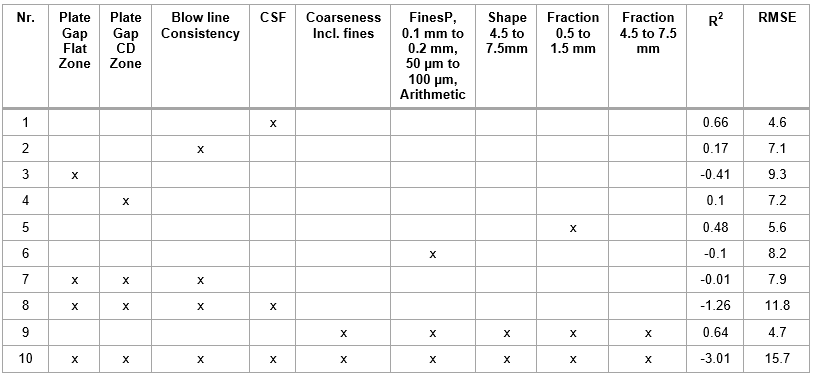Ekbåge, D., Nilsson, L., Håkansson, H., and Lin, P.-I. (2020). "Multiple linear regression modelling of pulp and handsheet properties based on fiber morphology measurements and process data," BioRes. 15(1), 654-676.

#### Abstract

A multiple regression model was evaluated to predict pulp and handsheet properties including z-directional tensile strength (z-strength) and Scott bond values. One hypothesis that was central for the model evaluation was that the crill content, as measured with ultraviolet and infrared lights, would improve the statistical models. A chemi-thermomechanical pulp (CTMP) mill designed with two parallel primary refining lines and a reject refiner was the basis for this study, and all process data and pulp samples were gathered from the specific process. Pulp was extracted from the process for an extended period from a position after the latency chest (primary refined pulp) and from the pulp-stream exiting the mill to the board machine (accept pulp). The crill content was positively correlated to the z-strength of the accept pulp, explaining 55% of the variance with a linear regression model with the crill content as the sole predictor. The estimation model of the z-strength of accept pulp was based on a combination of the crill content, freeness, fibril perimeter for longer fibers, and mean kink angle, and resulted in an R2 of 0.79. When applying cross-validation to determine the predictive model performance, the highest R2 obtained was 0.67. This latter model included the crill content, fibril perimeter, and mean kink angle as predictors.

Multiple Linear Regression Modelling of Pulp and Handsheet Properties Based on Fiber Morphology Measurements and Process Data

Daniel Ekbåge,a,* Lars Nilsson,a Helena Håkansson,a and Ping-I Lin b

A multiple regression model was evaluated to predict pulp and handsheet properties including z-directional tensile strength (z-strength) and Scott bond values. One hypothesis that was central for the model evaluation was that the crill content, as measured with ultraviolet and infrared lights, would improve the statistical models. A chemi-thermomechanical pulp (CTMP) mill designed with two parallel primary refining lines and a reject refiner was the basis for this study, and all process data and pulp samples were gathered from the specific process. Pulp was extracted from the process for an extended period from a position after the latency chest (primary refined pulp) and from the pulp-stream exiting the mill to the board machine (accept pulp). The crill content was positively correlated to the z-strength of the accept pulp, explaining 55% of the variance with a linear regression model with the crill content as the sole predictor. The estimation model of the z-strength of accept pulp was based on a combination of the crill content, freeness, fibril perimeter for longer fibers, and mean kink angle, and resulted in an R2 of 0.79. When applying cross-validation to determine the predictive model performance, the highest R2 obtained was 0.67. This latter model included the crill content, fibril perimeter, and mean kink angle as predictors.

Keywords: CTMP; Fiber morphology; Multiple regression modelling; Handsheet; Z-strength; Scott bond; Crill

Contact information: a: Department of Engineering and Chemical Sciences, Karlstad University, SE-651 88 Karlstad. Sweden; b: Department of Health Sciences, Karlstad University, SE-651 88 Karlstad;
* Corresponding author: daniel.ekbage@kau.se

INTRODUCTION

To gain knowledge of the resulting pulp properties of refined wood chips and relate these to current specifications (required properties), it is crucial to have a sensor collecting the pulp data in an accurate and fast manner. This information can be used to control the refining process. The relationship between the pulp properties and handsheet properties is important for improvements concerning energy efficiency and product quality. For decades there has been a need to fully understand the relationship between fiber characteristics and handsheet or paper properties. Some of the listed literature below deal with bonding and fiber strengths, concepts of load transfer, fiber distributions, impact of fibrillation, and influence of hemicellulose and cellulose compositions. Incorporation of the process data has also been studied, considering multiple temperature measurements in the refining zone for modelling purposes.

Page (1969) developed theories for the tensile strength of paper and postulated that the paper strength was dependent on both the tensile strength of the fibers and the bonds between the fibers. He developed an equation for the tensile strength of paper based on fiber and network properties. The equation declares that in the case of weakly bonded paper, the strength is controlled by the bonding strength. For papers with increasing strength, the fiber strength becomes important (Page 1969).

Another approach to predict the tensile strength of paper is the shear-lag, as outlined by Carlsson and Lindström (2005). This model is based on a concept of load transfer from the surrounding matrix to the fibers. The axial fiber stress is introduced via shear stresses acting at the fiber-matrix interface. If the fibers are short, then they will not reach the ultimate axial stress value before they are pulled out of the matrix. The analysis also indicates the importance of fiber length for weakly bonded sheets and also the relevance of relative bonded area for utilizing the strength potential of fibers.

Reyier Österling (2015) studied the distributions of fiber characteristics and how this information could be used to optimize the mechanical pulping process in terms of reducing the energy usage. The term bonding indicator (BIN) was introduced and defined as the bonding ability influence, which represented the predicted tensile index. If the BIN model is employed to predict the tensile index of the sheet, then it is recommended to use wall-volume-weight data. The results from Reyier Österling (2015) also revealed that the fraction of long fibers was important for the properties of the whole pulp, and that fiber wall thickness and external fibrillation were the fiber characteristics that contributed the most to tensile index of the long fiber fractions in the mechanical pulps investigated. Reyier Österling et al. (2012) studied the fiber dimensions impact on the tensile index and density of long fiber laboratory sheets. The results showed that the fibrillation index had a high positive influence on the long fiber tensile index and increased density, while the fiber width, fiber wall thickness, and collapse resistance were negative.

Tensile strength and Scott bond are strongly influenced by internal fibrillation and also external fibrillation (Kang and Paulapuro 2006). Karlström and Hill (2017) outlined a method that made it possible to model the Scott bond, z-strength, and tensile index for chemi-thermomechanical pulp (CTMP). Through the temperature profile measurements in the refining zone, they derived consistency and fiber residence time, and used these parameters as predictor variables in multilinear models. Models based on these types of internal variables outperformed models based on readily measurable process-variables as dilution water and plate clearance.

Through comparing various types of tests to measure the internal bond strength of papers, it has been observed that the z-directional tensile strength is the best parameter to determine the internal bond strength (Koubaa and Koran 1995). In this study the term z-strength is used to label a briefer form of this quantity.

The influence of hemicellulose and cellulose contents of spruce kraft pulp were investigated, and it was found that the Scott bond and z-strength were directly related to the sheet density and thus not influenced by the contents of hemicellulose and cellulose (Molin and Teder 2002).

The traditional method to determine the pulp characteristics involves the preparation of handsheets and the use of physical testing of the sheets. However, recently there has been a rapid development of devices for measuring the properties of fibers and pulp suspensions (Heikkurinen et al. 2009).

A widely-used indication of the pulp quality is freeness (Tervaskanto et al. 2009), and this property is a measure of the rate at which a dilute suspension may be drained according to TAPPI T227 om-17 (2017). There are also literature reports that deal with the small particles in the pulp, such as fines and crill particles (and how this property is measured), and online measurements for strength calculations.

According to TAPPI T261 cm-94 (1994), which outlines a standard method to measure the fines content of pulp for paper manufacture, the fines content is measured using a single-screen classifier. The pulp is characterized by dividing its content into particles labelled as ‘fibers’ and ‘fines’. A separation point of these two are the particles that pass through a round hole 76 µm in diameter or a 200-mesh screen as per the TAPPI T261 cm-94 (1994) standard. According to Odabas et al. (2016), the fines are a key issue in paper making, and their small size is the main property generating high specific surface area. The aforementioned study proposed the potential use of fines as a control variable to obtain desired paper properties. The fines are often split into fibrils, ray cells, and flake-like fines and the fibrillar material produced in mechanical pulping contributes to higher paper strength; the fine fibrillar material also leads to poor dewatering during the sheet-forming process. The fibrillar material produced during the pulping is also the fraction demonstrating the highest swelling in water (Odabas et al. 2016).

The addition of fines improves paper strength properties via consolidation between fibers (Bäckström et al. 2008). Chinga-Carrasco (2013) studied microfibrillated cellulosic (MFC) materials and considered fibrillation as the disintegration of cellulosic fibers into microfibrils. Through the use of optical techniques, this quantification can potentially be implemented in online production lines for process control (Chinga-Carrasco 2013). Light transmittance is considered a measure of the degree of fibrillation (Iwamoto et al. 2008; Chinga-Carrasco 2013). Chinga-Carrasco (2013) exemplified this on MFC films and showed that the light transmittance decreased with increased residual fiber fraction. In other words, a higher amount of fibrillar material present induced higher light transmittance (Chinga-Carrasco 2013). This work also included flowing MFC material through a transparent tube and taking images of the dynamic suspension with a camera system, for the determination of fibrillation degree. The aforementioned experiment confirmed that the light transmittance followed an equal trend as when it was measured with an optical scanner and ultraviolet-visible spectrophotometer.

Osong et al. (2014) studied the mechanical properties of handsheets of chemi-thermomechanical pulp (CTMP) mixed with nanolignocellulose and nanocellulose. Two of the conclusions were that the strength properties were increased via the addition and that it only had a slight effect in relation to the sheet density.

According to Lundberg et al. (2018), there is no formal definition for crill, which relates to the crill method that was outlined as the online measurements of pulp particles in the ultraviolet and infrared spectral regions. However, these particles are supposed to be the smaller fraction of fines (Lundberg et al. 2018). The aforementioned study claims that conventional image analysis methods can track changes in the fiber length and width, but not of the crill attached to fibers and/or free in the suspension. Moreover, the fibrillation index measured with a camera is limited by the image resolution, and thus only includes the fibrils attached to the fibers. Within the pulp and paper industry the manufacture of MFC and nanofibrillated cellulose is rapidly growing, and the crill method has been used for characterization (Lundberg et al. 2018).

Williamson and Back (2014) stated that important strength properties can be calculated from online measurements, thus contributing to stable pulp quality, quicker grade changes, and reduced laboratory work. They labeled the fine material as crill and described these particles as typically 0.25 μm in width, close to a hundred times thinner than fibers, and demonstrated that the high surface area associated with the crill is important for the strength properties of the pulp or the paper (Williamson and Back 2014). In a study made by Lindström and Aulin (2014), crill is characterized by very high anisotropy and a typical width of 0.1 µm to 1.0 µm.

In a study by Pettersson (2010), the crill was described as very thin particles that are completely or partially loosened from the fibers and being 100 times thinner than the fibers. The crill is measured via the comparison of two optically measured surface areas using light of various wavelengths. It reflects the degree of refining and is useful for troubleshooting, process analysis, monitoring, and control (Pettersson 2010).

The wavelength of the first light is comparable to the crill particle diameter (preferably ultraviolet light), and the second light is a wavelength longer than the first wavelength but shorter than the mean pulp diameter through the pulp suspension (Karlsson and Pettersson 1985).

With reference to the previously outlined literature, this paper adds value by including full-scale process and pulp data extracted for an extensive time period. The novelty is primarily given in the use of the statistical method for feature selection, model validation, combination of data, and measurement using ultraviolet (UV) and infrared (IR) lights for the specific pulp.

Physical Properties of Laboratory Sheets

Through measurement of the physical properties of the laboratory paper sheets, the relationship between the pulp data and the sheet properties can be investigated. In this work, the z-direction tensile strength and Scott bond were selected as responses as they are important for the final product properties. The z-direction tensile strength was obtained using a procedure for measuring the internal fiber bond strength for specimens subject to a normal separating force as per TAPPI T541 om-10 (2010) standard. The Scott bond is the most common test method for the determination of the delamination resistance of paper and board (Fellers et al. 2012) and measures the energy required to rapidly delaminate a specimen as per TAPPI T569 pm-00 (2000) standard.

The CTMP is often applied in the middle layer(s) in liquid packaging for providing a product structure with high stiffness, bulk, and good dimensionality (Lindholm et al. 2009).

The Specific CTMP Process

A specific CTMP mill designed with two parallel primary refining lines and a reject refiner was the basis for this study, and all of the process data and pulp samples were gathered from the specific process. The pulp samples were extracted from the process for an extended period, collected from a position after the latency chest, and labelled as primary refined pulp (Fig. 1); the pulp sample from the pulp-stream exiting the mill to the board machine was labelled as accept pulp (Fig. 2). The primary refined pulp was refined with a conical disc (CD) refiner followed by a fiber accelerator (driven by an electrical motor) for separation of the steam from the fibers (Fig. 1).

The raw material used for the CTMP process was spruce wood originating from a chip-line with logs and saw-mill chips.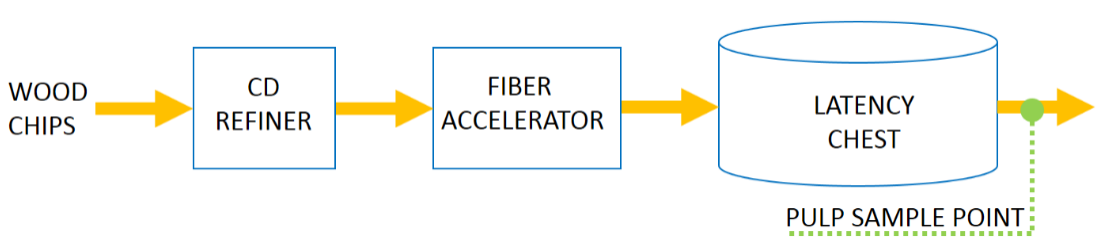Fig. 1. Simplified process schematic displaying sample point for primary refined pulp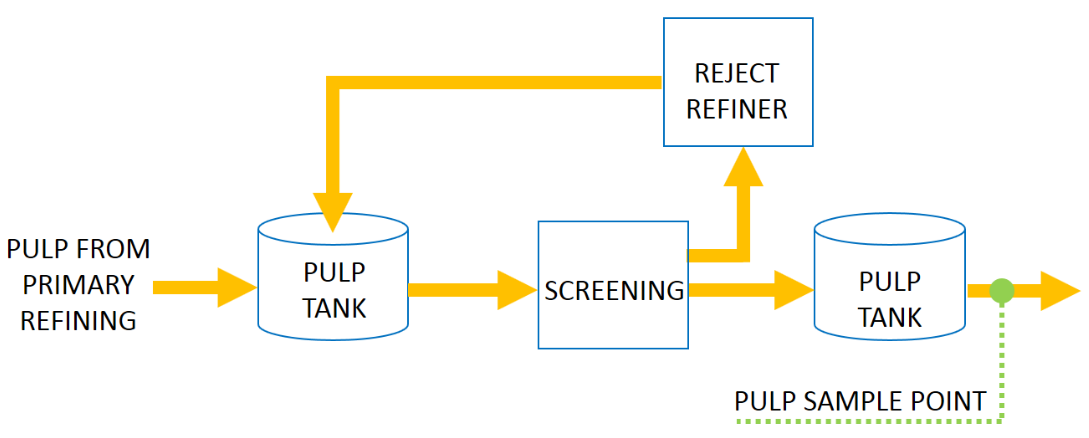Fig. 2. Simplified process schematic displaying sample point for accept pulp

The primary aim of this study was to evaluate the applicability of a multiple regression model for predicting the z-strength and Scott bond of laboratory handsheets prepared from CTMP extracted at two individual sample points; one representing the primary refined pulp and the second sample representing the accept pulp. This study has included the primary refined pulp to evaluate prediction early in the process, and the accept pulp is important as it is the final pulp mill product delivered to the board machine.

Multiple predictor variables were extracted from the image analysis of pulp samples with the addition of UV and IR lights to include crill content. The predictor variables further include freeness as well as process data for one of the pulps. The goal was to increase the understanding of the relationship between the pulp data and the resulting physical properties of laboratory sheets to support energy efficiency and product quality improvements, with a primary aim to reduce variability.

EXPERIMENTAL

Materials

Pulp preparation and measurements

During an extended period (close to 8 weeks for the total period), the pulp samples were extracted and afterwards stored in a refrigerator. Approximately 15 samples of primary refined pulp and 20 samples of accept pulp were used because they were considered to be representative and within normal mill operating conditions. No additives were added to these samples pulp because the pulp should be representative for the normal conditions. The fiber properties were analysed using an L&W fiber tester plus (ABB AB/Lorentzen & Wettre, Kista, Sweden) equipped with an L&W crill module (hence labelled ‘plus’). The crill-value in this study is measurement of light attenuation. This is done by using a UV-light source with a wavelength of 365 nm and a IR-light source with a wavelength of 850 nm. The light is sent through the pulp suspension, and the small particles will scatter and absorb light from the UV-light to a larger extent compared to the IR light. By comparison, the larger fibers will influence the light coming from the IR-light source more. The crill-value (which is assumed to be related to the content of crill) is calculated based on the comparison of the outgoing light from the UV-light source and the IR-light source (technical documentation, provided by ABB AB/Lorentzen & Wettre). Handsheets were prepared according to a procedure aligned with the methods used at the specific mill laboratory, to mimic the conditions. Preparation of the accept pulp prior to making laboratory sheets was initiated with a disintegrator set to 10,000 revolutions using close to 1300 g of pulp that had been diluted with approximately 2.5 L of non-heated water. This pulp was then diluted with 14 L of water in a container with pneumatic fiber stirring. Approximately 1000 g of the disintegrated pulp suspension was used for preparing a test sheet to determine the dry content. A manually operated sheet former with a circular grid plate and a pneumatic stirrer (model P15490; Messmer Büchel, Gravesend, United Kingdom), for fiber distribution was used for the manufacture of handsheets. The test sheet was dried in an L&W rapid dryer (ABB AB/Lorentzen & Wettre, Kista, Sweden) to determine the dry weight that was used to calculate the sample size from the diluted pulp, with a target grammage of 150 g/m2. After sheet forming, the sheets were pressed in two sequences in an L&W sheet press (type 94241; ABB AB/Lorentzen & Wettre, Kista, Sweden). A total of 5 handsheets were placed between circular steel plates and circular paper sheets. The first press sequence was set to create a pressure of 400 kPa in 5 min. The handsheet package was turned over, and new circular sheets were applied, followed by a final pressure sequence for 2 min with equal pressure. The z-strength was measured in a Zwick/Roell testing machine (type z005; Zwick/Roell, Ulm, Germany), equipped with a fixture for the z-directional tensile test of paper that conformed to TAPPI T541 om-10 (2010). Five quadratic pieces from the handsheet were applied in the z-strength fixture. For measurement of the Scott bond, an internal bond tester (model b; GCA/Precision Scientific Group, Chicago, IL, USA) was used and calibrated to operate in the low range (i.e., without the extra weights on the end of the pendulum). Additionally, in this case, 5 pieces from the handsheet were applied.

Freeness was measured according to the Scandinavian Pulp Paper and Board Testing Comittee (SCAN C21:65 1965) for all pulp samples. The data from the L&W Fiber Tester Plus included 49 variables describing fiber properties. For the accept pulp, these were the selected predictor variables. In all, 20 observations were made. Because the primary refined pulp samples could be synchronized with refiner process data, the predictor variables in this analysis included L&W Fiber Tester Plus data (49 parameters), process data, and freeness (18 variables) for 15 observations. The process data were synchronized with the sample time of the pulp, taking lag times into account, for example the hydraulic residence time in the latency chest and lag for changes in chip mix (ratio of saw-mill chips to the chips from logs).

Methods

Repeatability test of crill in fiber analyser

To determine the repeatability of the crill measurement, a 10-sample test was made in the L&W fiber tester plus analyser with the pulp originating from one pulp sample.

Repeatability was determined by calculating one standard deviation of the 10 pulp samples. This was calculated for both the primary refined pulp and accept pulp.

Process data

For the study of primary refined pulp, a number of refining process data variables were combined with the L&W fiber tester plus measurements, as input to the statistical analysis. The process data variables were production, plate gap in flat zone, plate gap in CD zone, water dilution to flat zone, water dilution to CD zone, water dilution temperature, water dilution pressure, refiner power, specific electricity consumption, refiner housing pressure, pre-heater pressure, temperature in flat zone, temperature in CD zone, blow line consistency, and the ratio of saw mill chips to the log chips. To enable synchronization between process and pulp data, all process variables were synchronized with the timestamp of the pulp sample.

Modelling techniques

Variable selection in statistical analysis is the key to the identification of important variables in relation to their explanatory effect on a dependent variable. There are several selection techniques available and the one used in this study was Lasso (least absolute shrinkage and selection operator).

The Lasso is a method for estimation in linear models. It minimizes the residual sum of squares subject to the sum of the absolute value of the coefficients being less than a constant, providing the ability to produce coefficients that are exactly zero, which gives interpretable models (Tibshirani 1996).

This technique can be explained starting with the normal linear regression modelling, introducing d as the dependent variable, as a matrix containing the independent variables, and β as the model coefficients. The following Eq. 1 defines the relationship in a given set of data: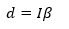(1)

For the ordinary least square approach, Eq. 2 outlines the procedure for minimizing the sum of squares of the prediction errors: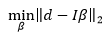(2)

Regularization is a method within statistical modelling to prevent overfitting. The estimation of this parameter is also known as a shrinkage technique, and is an alternative to variable selection methods (e.g., stepwise regression) and data reduction techniques (e.g., principal component regression) (Finch and Finch 2016).

For the Lasso method, a regularization term is added to Eq. 2,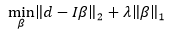(3)

where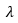is a parameter that the algorithm locates at the minimum error. Aschanges, different levels of regularization generate the models. Overall, the concept places penalty in retaining many non-zero coefficients and punishes large coefficients.

To summarize, the features characterizing Lasso are: 1) Reducing the number of predictors (by generating zero-valued coefficients); 2) Identifying important predictors; 3) Selecting amongst redundant predictors; and 4) Generating shrinkage estimates with potentially lower errors than the ordinary least squares method (MATLAB and Statistics and Machine Learning Toolbox Release 2017b, The MathWorks, Inc., Natick, MA, USA).

In this study, the statistical modelling and analysis was performed using MATLAB R2017b (The MathWorks, Inc., Natick, MA, USA) and R version 3.5.1 (R Foundation for Statistical Computing, Vienna, Austria).

Table 1. L&W Fiber Tester Plus Parameters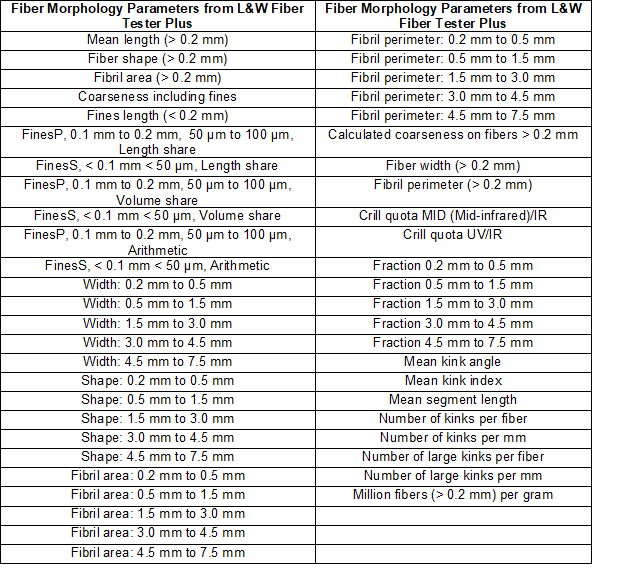Multiple linear regression is a technique for the estimation of models to fit data from several variables given one response variable. A multiple regression model was defined with n variables, as I1I2, …, In and the dependent (response) variable D is written as,

D = β0+ β1 I1+ β2 I2+… βn In +ε (4)

where ε is the residual terms of the model. The quality of the estimation and validation models in this study were determined via calculation of the R2 (coefficient of determination) and the root mean squared error (RMSE).

R2 is defined as (Mathworks 2017a),

R2 = 1 – SSE/SST (5)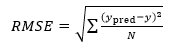(6)

where R2 is the coefficient of determination, SSE is the sum of squared error, and SST is the sum of squared total (Mathworks 2017a). The RMSE is the root mean squared error, is the predicted value, is the actual value, and is the number of observations. When Eq. 5 was used in the model validation part in MATLAB, the results for a bad model fit can be negative values, indicating model overfitting.

The statistical models were divided into estimated and validated models. An estimated model means that it has not been evaluated against test data, whereas a validated model has been generated with a training data set and then validated with test data set (which was not part of the observations used for the model generation).

Fiber Morphology Parameters from L&W Fiber Tester Plus

The L&W fiber tester plus generated a total of 49 parameters from each pulp sample, mainly describing morphology. Some of this data represent the fiber dimensions, distributions, shape, and crill (Table 1).

RESULTS AND DISCUSSION

Repeatability Test of Crill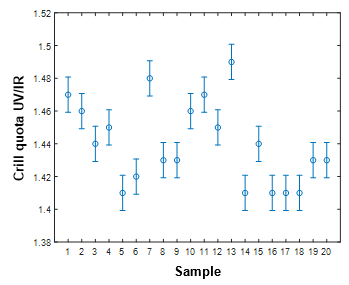Fig. 3. The 20 observations of crill measurements with error bar calculated from the repeatability test

The standard deviations of the accept pulp and primary refined pulp were 0.011 and 0.018, respectively, revealing a better repeatability for the accept pulp.

Implementing this variability into the 20 observations for the accept pulp revealed the presence of significant differences (Fig. 3).

Physical Properties of Laboratory Handsheets

The results indicated a significant difference in the z-strength when comparing the accept pulp and the primary refined pulp, with a p-value of 3.4e-13 at the 5% significance level, given a null hypothesis of equal means. The median was 80.2 kPa for accept pulp and 46.6 kPa for primary refined pulp (Fig. 4).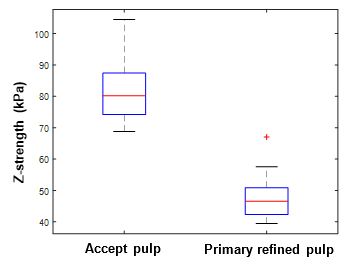Fig. 4. Boxplots of z-strength for accept pulp and primary refined pulp

The Scott bond (Fig. 5) was also significantly different for the two pulp qualities, indicating a p-value of 1.96e-14.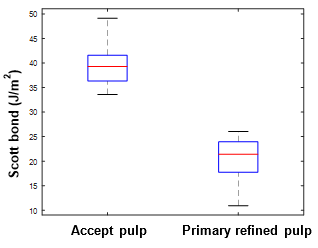Fig. 5. Boxplot of Scott bond for accept and primary refined pulp

The series of 20 observations of the z-strength of accept pulp (Fig. 6) exposed significant changes for a large amount of the samples and had feasible characteristics for the statistical modelling phase.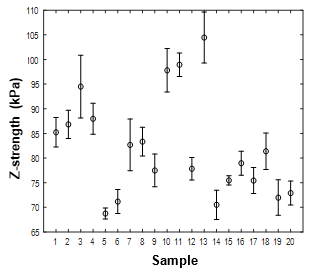Fig. 6. Z-strength for accept pulp with error bar representing one standard deviation of five measurements

It was observed that the Scott bond measurements did not vary significantly along the series of samples, so that Scott bond was not a part of the statistical modelling procedure. The arguments for not including the Scott bond measurements were that the variations in most of the individual samples were high, which generated difficulties to identify significant process variations in the response variable. Figure 7 shows the Scott bond measurements for the primary refined pulp.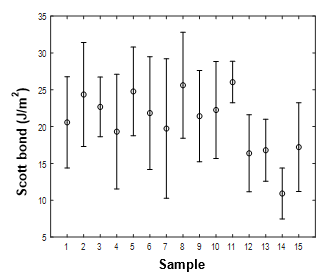Fig. 7. Scott bond for primary refined pulp, including error bar representing one standard deviation of five measurements

Feature Selection and Model Estimation of Z-Strength for Accept Pulp

Through applying the Lasso regression to the dataset with the z-strength as the dependent variable, the following variables were selected: Canadian Standard Freeness (CSF), fibril perimeter, crill, and mean kink angle. The mean kink angle and CSF had negative correlation coefficients with the z-strength, whereas the fibril perimeter and crill were positively correlated to the z-strength (Fig. 8). CSF had a negative correlation to crill, and fibril perimeter had a positive correlation to crill. These results were found reasonable since it was expected that the amount of fines increases with increased refining, and crill increases with increased fibrillation.

The correlations are displayed with correlograms that provide a specific area with color representing the value of the correlation coefficient. Red color means negative correlation and blue indicates positive correlation, and a higher color intensity designates a higher correlation. A larger area of the square means a higher correlation coefficient was obtained.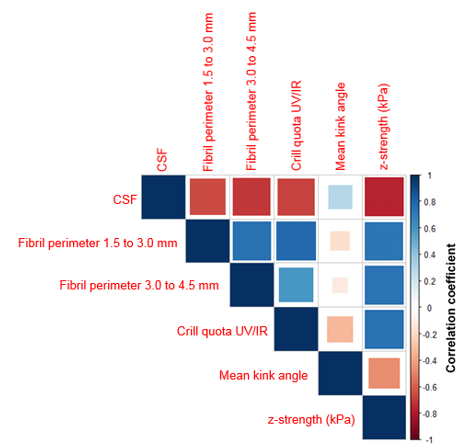Fig. 8. Correlogram for variables selected with z-strength as dependent variable

As the number of observations was limited, a multiple regression model including fewer independent variables than the entire list was studied. The model estimation revealed that a model based on fibril perimeter, crill, and kink angle gave an R2 value of 0.77 (Table 2 and Fig. 9b), indicating that 77% of the variance in the z-strength could be explained by these four predictor variables.

Table 2. Z-strength Estimation Models for Accept Pulp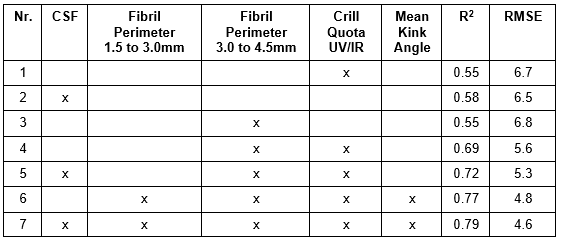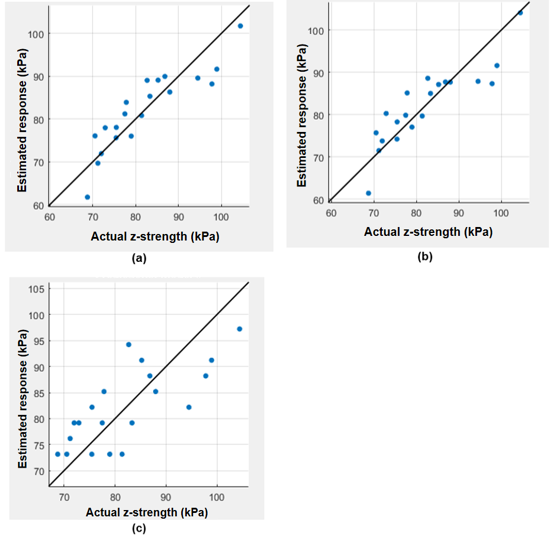Fig. 9. Z-strength estimation models, based on data for accept pulp: (a) includes CSF, fibril perimeter, crill, mean kink angle; (b) includes fibril perimeter, crill, mean kink angle; and (c) crill

The addition of CSF to the model improved the R2 to 0.79 (Fig. 9a). If only the crill or the CSF variable was present in the model then a R2 = 0.55 (Fig. 9 c) was observed. The R2 increased with the number of predictor variables and the model errors were decreased.

Model Validation of Z-strength for Accept Pulp

The validation process, based on 5-fold cross validation, indicated that the model including crill, fibril perimeter, and mean kink angle, had the highest R2 of the validated models at 0.67 (Table 3 and Figs. 10a and 10b).

Table 3. Z-strength Validation Models for Accept Pulp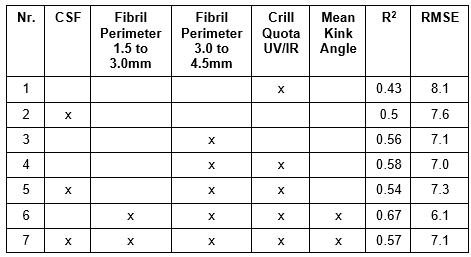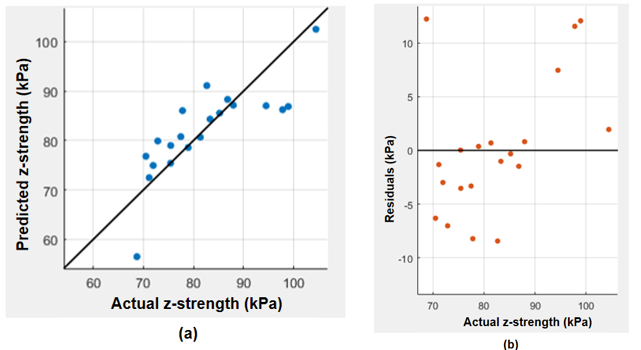Fig. 10. Z-strength validation model based on data for accept pulp: Fibril perimeter, crill, and mean kink angle, showing the predicted vs. actual values (a) and residuals (b)

If the model contained only the crill variable, 43% of the variance was explained, which can be compared to the estimation model at 55%. It was observed that the addition of CSF to the fiber and crill variables reduced the R2 value from 0.67 to 0.57.

Based on the tabulated results of the estimation and validation models, it was observed that the RMSE developed differently with the model complexity (number of variables present in the statistical model). The RMSE of the estimation model decreased with increased model complexity over the entire range of predicting variables used, whereas the validation model indicated a minimum RMSE at a model complexity of 4 (Fig. 11), which in this case represented fibril perimeter, crill, and mean kink angle.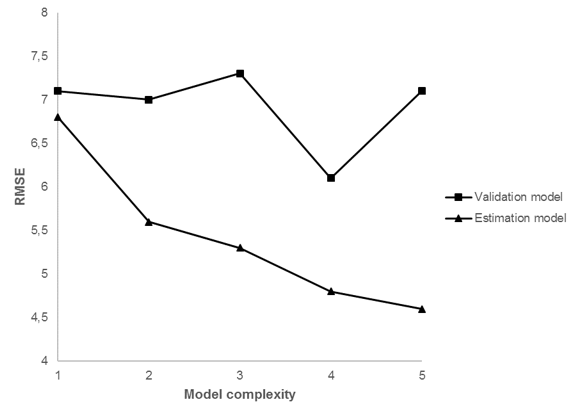Fig. 11. Model complexity and RMSE for estimation and validation models

Model Estimation and Feature Selection of Z-Strength of Primary Refined Pulp

For the z-strength of primary refined pulp as the dependent variable, Lasso selected plate gaps, blow line consistency, CSF, coarseness, fines, shape, and smaller and larger fractions (Fig. 12). The highest negative correlation coefficient was observed for CSF, and the highest positive correlation was with fraction 0.5 mm to 1.5 mm. The single variable that explained most of the variance was CSF (Table 4), with an R2 of 0.77. The fraction at 0.5 mm to 1.5 mm was second highest at R= 0.7. The process variables only reached an R2 value of 0.61 and through the addition of CSF this value was increased to 0.91. The Rof fiber morphology only reached 0.92. Including all variables in the linear regression model resulted in an R2 of 0.95 (Table 4).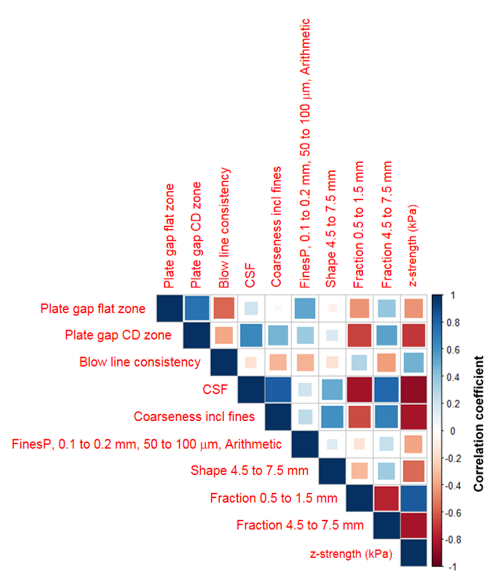Fig. 12. Correlogram for variables selected having z-strength as dependent variable

Correlation Analysis of Process and Fiber Morphology Data Based on 27 Observations of the Primary Refined Pulp

The correlation study of process and L&W fiber tester plus data based on 27 observations of the primary refined pulp is displayed in Fig. 13. The correlogram indicates that crill content had a negative correlation with the refiner gap and the quota of saw mill chips. One of the highest positive correlations was obtained with refiner power. Dilution water in the CD zone indicated a significant negative correlation to the fiber shape and width, whereas the flow in the flat zone was positively correlated to the fiber shape. The mean length was significantly positively correlated to the plate gap in the CD zone and negative to the refiner power and refiner pressure.

Table 4. Z-strength Estimation Models for Primary Refined Pulp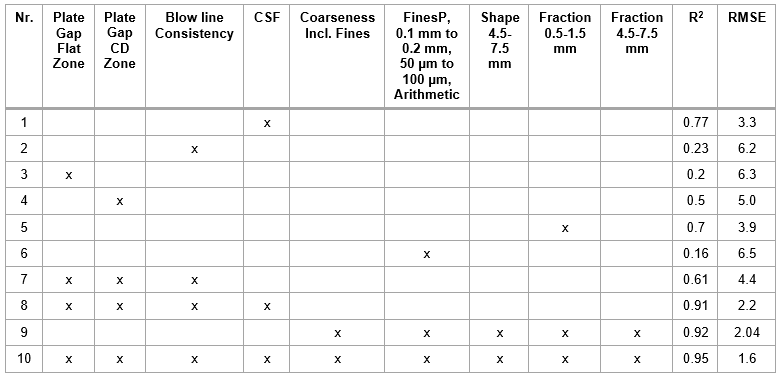Model Validation of Z-strength of Primary Refined Pulp

These results varied a lot depending on the predictor variables selected (Table 5). For the validated models, the one with the highest R2 included only the fiber morphology and no process variables and had an R2 of 0.64. Including the CSF variable only improved the R2 to 0.66. Most of the models had very low or negative R2 values.

Table 5. Z-Strength Validation Models for Primary Refined Pulp